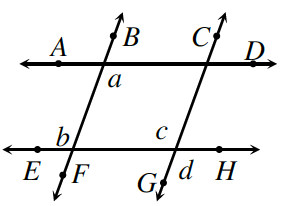### Home > INT2 > Chapter 7 > Lesson 7.1.3 > Problem7-39

7-39.

Penn started the proof below to show that if $\overline{AD} // \overline{EH}$ and $\overline{BF} // \overline{CG}$, then $a = d$. Unfortunately, he did not provide reasons for his proof. Copy his proof and provide a justification for each statement.

Statements

Reasons

1. $\overline{AD} // \overline{EH}$

1. $a = b$

1. $\overline{BF} // \overline{CG}$

1. $b = c$

1. $a = c$

1. $c = d$

1. $a = d$For each numbered statement, write how you know it to be true. Think about how angles and parallel lines relate to each other.

Justification for statement 1: Given

Justification for statement 2: alternate interior angles are congruent